Courses

# Doc: Moving Coil Galvanometer Class 12 Notes | EduRev

## Class 12: Doc: Moving Coil Galvanometer Class 12 Notes | EduRev

The document Doc: Moving Coil Galvanometer Class 12 Notes | EduRev is a part of the Class 12 Course Physics For JEE.
All you need of Class 12 at this link: Class 12

18. MOVING-COIL GALVANOMETER: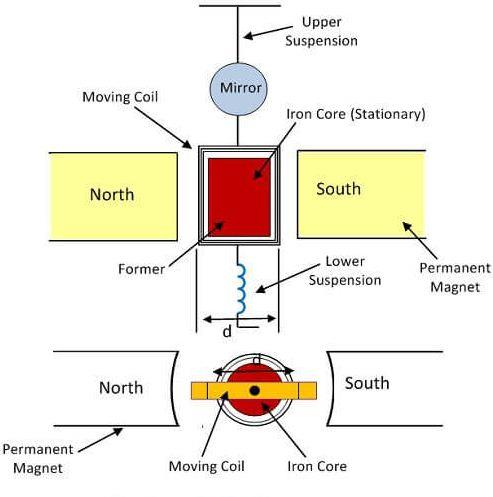Fig: Moving coil galvanometer

The main parts of a moving-coil galvanometer are shown in figure. A rectangular coil of several turns is wound over a soft-iron core. The wire of the coil is coated with an insulating material so that each turn is insulated from the other and from the iron core. The coil is suspended between the two pole pieces of a strong permanent magnet.

The current to be measured is passed through the galvanometer. As the coil is in the magnetic field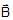of the permanent magnet, a torque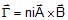acts on the coil. Here n = number of turns, i = current in the coil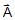= area-vector of the coil and= magnetic field at the site of the coil. This torque deflects the coil from its equilibrium position.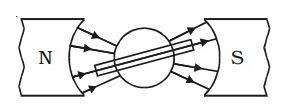Fig: Radial magnetic field

The pole pieces are made cylindrical. As a result, the magnetic field at the arms of the coil remains parallel to the plane of the coil everywhere even as the coil rotates. The deflecting torque is then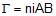. As the upper end of the suspension strip W is fixed, the strip gets twisted when the coil rotates.

This produces a restoring torque acting on the coil. If the deflection of the coil is q and the torsional constant of the suspension strip is k, the restoring torque is kq. The coil will stay at a deflection q where:

niAB = kθ or,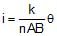Hence, the current is proportional to the deflection. The constant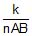is called the galvanometer constant, and may be found by passing a known current, measuring the deflection q and putting these values in equation.

Sensitivity:

The sensitivity of a moving-coil galvanometer is defined as θ/i. From equation, the sensitivity is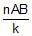. For large sensitivity, the field B should be large. The presence of soft-core increases the magnetic field.

The document Doc: Moving Coil Galvanometer Class 12 Notes | EduRev is a part of the Class 12 Course Physics For JEE.
All you need of Class 12 at this link: Class 12Use Code STAYHOME200 and get INR 200 additional OFF Use Coupon Code
All Tests, Videos & Notes of Class 12: Class 12## Physics For JEE

187 videos|516 docs|263 tests

Track your progress, build streaks, highlight & save important lessons and more!

,

,

,

,

,

,

,

,

,

,

,

,

,

,

,

,

,

,

,

,

,

;